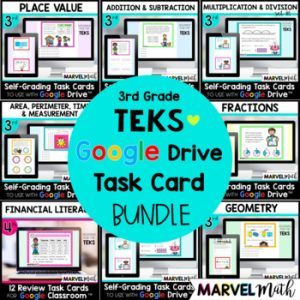Save 10% on your first order with code EXTRA10

\$4.00\$151.25\$23.50
SKU: 5561566 Categories: ,

## Description

This resource includes 24 TEKS-aligned Digital Task Cards for Fractions that can be assigned for Distance Learning via Google Classroom. Topics covered include fractions on a number line, equivalent fractions, comparing fractions, and reasoning about fractions. These task cards are in a Google Form, which means they are automatically graded when students click submit!

I have designed these task cards to give more weight to the Readiness Standards which comprise nearly 2/3 of the STAAR test. The questions are rigorous and have bright graphics to keep students engaged. All questions are multiple choice.

This resource can be used as a 24 question review activity, but it can also be used in many other ways! You can make a copy of the Google Form and delete questions to differentiate. There are 24 questions included, so you also have the ability to create multiple reviews from this resource, splitting up the questions to use in a variety of ways. Please note that the questions and answers are not editable.

You do not need Google Classroom to use this resource, but you must have a Google e-mail to use Google Forms.

This set includes Task Cards to help students practice all of the Fraction TEKS.

What’s Included?

• Printable Teacher Tracking sheet to record names of students who are struggling with each of the TEKS (based on the problem numbers they missed)

Topics include:

• Representing Fractions with Models
• Fractions on a Number Line
• Explaining Unit Fractions
• Representing Equivalent Fractions with Models and on Number Lines
• Comparing Fractions
• Composing and Decomposing Fractions into unit fractions (3/4 = 1/4 + 1/4 + 1/4)
• Dividing Objects into Fractional Shares

TEKS Alignment:

3.3F: (5 questions) Represent equivalent fractions with denominators of 2, 3, 4, 6, and 8 using a variety of objects and pictorial models, including number lines.

3.3H: (5 questions) Compare two fractions having the same numerator or denominator in problems by reasoning about their sizes and justifying the conclusion using symbols, words, objects, and pictorial models.

Supporting Standards

3.3A: (2 questions) Represent fractions greater than zero and less than or equal to one with denominators of 2, 3, 4, 6, and 8 using concrete objects and pictorial models, including strip diagrams and number lines.

3.3B: (2 questions) Determine the corresponding fraction greater than zero and less than or equal to one with denominators of 2, 3, 4, 6, and 8 given a specified point on a number line.

3.3C: (2 questions) Explain that the unit fraction 1/b represents the quantity formed by one part of a whole that has been partitioned into b equal parts where b is a non-zero whole number.

3.3D: (2 questions) Compose and decompose a fraction a/b with a numerator greater than zero and less than or equal to b as a sum of parts 1/b.

3.3E: (2 questions) Solve problems involving partitioning an object or a set of objects among two or more recipients using pictorial representations of fractions with denominators of 2, 3, 4, 6, and 8.

3.3G: (2 questions) Explain that two fractions are equivalent if and only if they are both represented by the same point on the number line or represent the same portion of a same size whole for an area model.